# Bohr Model of the Atom Chemistry Tutorial

## Key Concepts

In 1913 Niels Bohr proposed a model for atomic structure based on the following postulates:

• Electrons moved around the nucleus in fixed orbits.
An electron in a particular orbit has a constant amount of energy.
• If an electron absorbs energy it moves to an orbit with a larger radius, that is, an orbit of greater energy.
The electron is said to be excited.
• If the electron falls back to an orbit with a smaller radius, one of lower energy, then the excess energy is emitted.
• The electron can only exist in certain discrete energy levels, therefore only particular orbits are available to it.
We refer to energy being quantised.

Limitations of the Bohr model of the atom. Bohr model of the atom does not explain:

• spectra of atoms or ions with more than 1 electron
• relative intensities of spectral lines in a spectrum
• hyperfine structure of spectral lines
• Zeeman effect

No ads = no money for us = no free stuff for you!

## Models of the Atom Before Bohr

Ernest Rutherford had proposed a nuclear model for the atom before Niels Bohr.
Rutherford's model of the atom:

• Nearly all the mass and the positive charge is concentrated in a small central nucleus.
• Electrons occupy the rest of the space, moving rapidly in circular orbits around the nucleus.
Coulomb attraction between negatively charged electrons and positively charged nucleus being just sufficient to supply the necessary centripetal force to keep the electrons in orbit.
• Most of the atom is empty space.

• An electron moving like this should be continually emitting light, losing energy, and spiralling into the nucleus.
According to the Rutherford model an atom should be unstable, but atoms are observed to be stable.

• The frequency of the light emitted should be changing continuously.
An element's emission spectrum would be a continuous spectrum like that shown below:

 Continuous Spectrum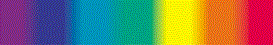The emission spectrum of elements is not a continuous spectrum, it contains a set of discrete lines.
The emission spectrum for hydrogen is shown below:

 Hydrogen Emission Spectrum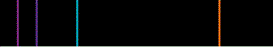Niels Bohr set out to propose a model of the atom to explain the stability and emission spectrum of atoms.

Do you know this?

Play the game now!

## The Bohr Model of the Atom

Niels Bohr applied the quantum concept of quantisation of energy, energy can only exist in discrete packets of energy, to Rutherford's classical model in order to explain the stability of the hydrogen atom and its discrete emission spectrum.

The Rutherford model predicted an unstable atom, one in which the negatively charged electron spiralled into the positively charged nucleus, emitting energy continuously.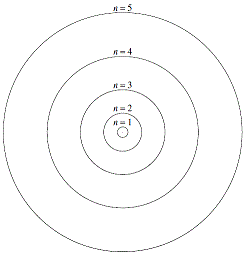Bohr overcame this difficulty by assuming a quantum description for energy transfer, and by stating that classical theory did not apply because atoms were stable. Electrons could only exist in particular fixed energy states which he called stationary states. An electron in this stationary state does not radiate energy.
The first five circular Bohr orbits or stationary states are shown on the right.
The small positively charged nucleus is shown as a point at the center of the diagram.
Each circle represents a possible circular orbit (stationary state) for an electron.
Each circular orbit (stationary state) has a particular energy and therefore a particular radius.
Energy increases as you move away from the nucleus.
Radius of the orbit increases as you move away from the nucleus.
The circular orbit labelled "n=1" closest to the nucleus has the smallest radius, and therefore the lowest energy.
In this diagram, the circular orbit (stationary state) labelled "n=5" has the greatest energy and the largest radius.

Bohr's model of the atom is referred to as a:

• planetary model: electrons circle the nucleus of an atom like the planets "circle" the sun
• nuclear model: there is a small central nucleus around which the electons orbit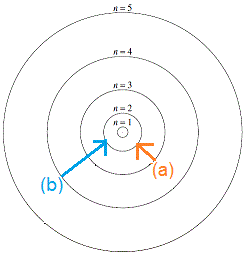According to Bohr's model of the atom, an electron could jump from one orbit to another, but it could not occupy the space between orbits.

The diagram on the right shows two possible quantum jumps an electron in a higher orbit could make to jump down to the n=2 orbit. The arrow shows the direction in which the electron jumps:

(a) electron in the n=3 orbit jumps down to the n=2 orbit

(b) electron in the n=4 orbit jumps down to the n=2 orbit

Bohr assumed that the Law of Conservation of Energy was valid so that energy could neither be created nor destroyed, but energy can be transformed from one type into another.
Therefore, if an electron had energy E3 in orbit n=3, when it falls down to the lower orbit n=2 it will have less energy, E2.
E3 > E2

Let E equal the energy difference between between E3 and E2:

E = E3 - E2

What is this new energy term, E?
E represents the amount of energy that is transformed when the electron falls from the n=3 orbit to the n=2 orbit.
But what is this energy transformed into?
This is the amount of energy transformed into a photon of light!
The energy (E) of a photon is given as Planck's constant (h) multiplied by the frequency (f) of the photon:
E = hf

So we can write
E = E3 - E2 = hf

Plank's constant, h, is a constant (6.626 × 10-34 J s), so we can say that the energy of a photon, E, is proportional to its frequency:
E ∝ f

This means that the greater the energy of the photon emitted, the greater its frequency will be.
The wavelength (λ) of a photon is inversely proportional to its frequency (f):
 f ∝ 1   λ

So we can see that the energy (E) of the photon emitted is also inversely proportional to its wavelength (λ):
 E ∝ 1   λ

In other words, a photon with lots of energy has a smaller wavelength, whereas a photon with less energy has a longer wavelength.

Remember that the electron can only exist in particular energy states (orbits) according to Bohr so only certain amounts of energy can be emitted when an electron jumps from a higher energy orbit to a lower energy orbit, therefore the wavelengths of light emitted will be restricted to those with this amount of energy.

Hydrogen, with just one electron, is the simplest atom and has the least complicated emission spectrum.
The hydrogen emission spectrum is made up of a series of lines of particular wavelength, four of which occur in the visible part of the electromagnetic spectrum as shown below:Each line in the emission spectrum corresponds to light of a particular wavelength, and in the visible part of the electromagnetic spectrum each of these wavelengths corresponds to a particular colour.

According to the Bohr model of the atom, each discrete line in the spectrum corresponds to the energy emitted when an electron jumps down from a higher energy stationary state (higher orbit) to a lower energy stationary state (lower orbit).

Do you understand this?

Take the test now!

## Evidence for the Existence of Stationary States

Bohr's model of the atom was successful in describing the hydrogen emission spectrum, but what would happen to the energy of an electron if you fired it at an atom?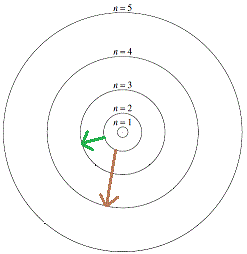According to Bohr, the energy of the incident electron should be absorbed in discrete amounts.
An electron in the n=2 orbit with energy E2, for example, could jump to the n=3 orbit with energy E3 by absorbing energy equal to E3 - E2 as shown by the diagram in green.
Similarly, an electron in the n=2 orbit could jump to the n=4 orbit with E4 energy by absorbing energy equal to E4 - E2 as shown by the diagram in brown.
If the incident electron had energy somewhere between E3 - E2 and E4 - E2, then only energy equal to E3 - E2 could be absorbed, and the scattered electron would exit the collision retaining the energy left after absorption by the atom.
That is, if an incident electron had kinetic energy, KEi, before collision with an atom, then the atom would absorb some of this energy on collision, E, and the electron would leave the collision with lower kinetic energy, KEf:

KEi = energy of indicident electron
KEf = energy of electron after collision with an atom
E = energy absorbed by atom during collision

KEi = KEf + E

KEi > KEf

So, if you fired electrons with similar but different kinetic energy at an atom, the atom should absorb the same amount of energy, E, from each incident electron if stationary states actually exist.
If stationary states do not exist, then the amount of energy absorbed by the atom will be different for each incident electron.

In 1914, James Franck and Gustav Hertz fired electrons at mercury atoms contained in mercury vapour at very low pressure in a chamber.
The kinetic energy of the bombarding electrons was observed both before and after being scattered by the mercury atoms.
Some of the results of their experiments are tabulated below:

 kinetic energy of incident electron / eV kinetic energy of scattered electron / eV difference in kinetic energy of electron / eV 1 5 5.5 1 0.1 0.6 0 4.9 4.9

A 1 eV1 low energy electron collided elastically with the large mercury atoms, no energy was absorbed by the atoms.
A 5 eV electron collided with a mercury atom and was found to have only 0.1 eV of energy after collision. So 4.9 eV of energy had been absorbed by a mercury atom.
Similarly, a 5.5 eV electron was found to have only 0.6 eV of energy after collision so that a mercury atom had absorbed 4.9 eV of energy.

Franck and Hertz concluded that there was a stationary state at 4.9 eV.
A mercury atom that absorbed 4.9 eV of energy would then be in an excited state.

What would happen to an electron in this excited state?
Franck and Hertz surmised that the excited electron would soon fall back to into the more stable, that is lower energy, ground state. In doing so, a photon of light would be emitted which would have energy equal to 4.9 eV. This amount of energy corresponds to a wavelength of 2.535 × 10-7 m or 253.5 nm and when they searched for this line they did indeed find it.

Further experiments showed that mercury atoms absorb other discrete amounts of energy, such as 6.7 eV and 10.4 eV, when bombarded with electrons.
This shows the existence of a number of different stationary states for the mercury atom, such as would be predicted by the Bohr model of the atom.
Other elements were also shown to have discrete absorption levels, and each corresponded with the wavelength of a line in its emission spectrum.

The absorption spectrum for hydrogen is shown below: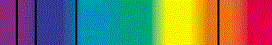The black lines in the absorption spectrum correspond to the energy, and therefore wavelengths, of photons of light that have been absorbed by the hydrogen atoms.
As you would expect, since only particular amounts of energy can be absorbed by an atom, the same particular amounts of energy can also only be emitted by the same atoms.
This means that the wavelengths of coloured light you see in the emission spectrum for hydrogen correspond exactly to the wavelengths of the black lines seen in the absorption spectrum for hydrogen:

 hydrogen emission spectrumhydrogen absorption spectrumCan you apply this?

Join AUS-e-TUTE!

Take the exam now!

## Successes of the Bohr Model of the Atom

Bohr's model of the atom successfully described the emission and absorption spectrum of hydrogen.
It could also predict the spectra of other one-electron atoms or ions such as:

• dueterium (heavy hydrogen, or hydrogen-2) whose spectrum is slightly different to hydrogen-1
• He+ (singly ionised helium formed when a helium atom loses 1 electron)
• Li2+ (doubly ionised lithium formed when a lithium atom loses 2 electrons)

With some modification, the Bohr model of the atom could also partially explain the spectra of atoms with 1 outer shell (valence) electron, such as the sodium atom.
The Bohr model of the atom was qualitatively successful at describing the spectra of atoms containing more than 1 outer shell (valence) electron.
That is, the description of a spectrum as an electron jumping from a higher energy orbit to a lower energy orbit and emitting a quantum of energy resulting in a spectral line of frequencey f and wavelength λ, works.

## Limitations of the Bohr Model of the Atom

• Quantitatively, the Bohr model of the atom could not cope with the spectrum of any atom other than hydrogen.
That is, the calculation of quantities only worked for the atom hydrogen.
• Bohr model could not be used to predict the intensity of a particular spectral line.
Some lines in a spectrum are more intense, that is brighter, than others. This means that there must be more electrons making one particular "jump" or transition. Therefore there will be more emissions of this particular wavelength making this line brighter than other lines in the spectrum. This is not predicted by the Bohr model of the atom.
• Bohr model could not explain the hyperfine structure of a spectrum.
Spectrometers with higher resolving power than that available in 1913 show that some spectral lines which were thought to be single lines turn out to be split into two lines (a doublet) or even three lines (a triplet). These lines are very close together, just nanometres apart.
• Bohr model of the atom could not explain the Zeeman effect.
The single line of a spectrum is split into pairs of lines with slightly different wavelengths when a strong magnetic field is applied to the vapour being investigated.

Can you apply this?

Join AUS-e-TUTE!

Take the exam now!

Footnotes:

1. Physicists often use the electron-volt (eV) as a convenient unit of energy. It is not an SI unit. The SI unit of energy is the joule (J).
1 eV = 1.602 × 10-19 J